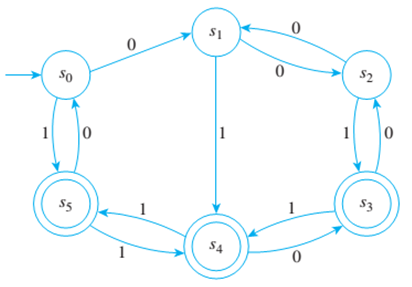Chapter 12.3, Problem 4ES### Discrete Mathematics With Applicat...

5th Edition
EPP + 1 other
ISBN: 9781337694193

#### Solutions

Chapter
Section### Discrete Mathematics With Applicat...

5th Edition
EPP + 1 other
ISBN: 9781337694193
Textbook Problem
1 views

# Consider the finite-state automaton given by the following transition diagram:a. Find the 0-, 1-, 2-, and 3-equivalence classes of states of A. b. Draw the transition diagram for A ¯ , the quotient automaton of A.

To determine

(a)

Find the 0,1,2and3 equivalence classes of states of A.

Explanation

Given information:

The transition diagram of a finite state automaton A is given as follows.

Concept used:

Arrow in the given graph played vital role in answer selection.

Calculation:

It is required to rind the 0,1,2 and 3 equivalence classes of states of A.

0 Equivalence classes - Two states are zero equivalent if both are accepting states or both are non-accepting states. Therefore, the 0 equivalence classes are: {s0,s1,s2},{s4,s3,s5}

1 Equivalence classes — Two states are 1 equivalent if, and only if they are 0 equivalent and, after input of any symbol, their next state are 0 equivalent. Therefore, the 1 equivalence classes are: {s0,s1,s2},{s3,s5},{s4}

2 Equivalence classes —two states are called 2 equivalent if, and only ¡f they are 1 equivalent and, after input of any symbol, their next state are 1 equivalent. Therefore the 2 equivalent classes are: {s0,s2},{s1},{s3,s5},{s4}

3 Equivalence classes — Two states are called 3 equivalent if, and only if they are 2 equivalent and, after input of any symbol, their next state are 2 equivalent

To determine

(b)

Draw the transition diagram of A¯ the quotient automaton of A.

### Still sussing out bartleby?

Check out a sample textbook solution.

See a sample solution

#### The Solution to Your Study Problems

Bartleby provides explanations to thousands of textbook problems written by our experts, many with advanced degrees!

Get Started